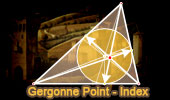# Online Geometry: Gergonne Points, Theorems and Problems - Table of ContentGergonne Point Theorem. Concurrency. Interactive proof with animation.
HTML5 Animation for Tablets (iPad, Nexus)
Key concept: Ceva's Theorem.

Geometry Problem 1024.
Contact, Gergonne Triangle, Point on an arc of Incircle, Perpendicular, Distances.

Geometry Problem 1023.
Triangle, Contact, Gergonne, Product of three distances.

Geometry Problem 821.

Geometry Problem 689.
Triangle, Three Excircles, Tangency points, Tangent lines, Concurrent Lines, Mind Map.

Geometry Problem 687.
Triangle, Three Excircles, Tangency points, Concurrent Lines
.

Geometry Problem 681: Gergonne Point.
Triangle, Incircle, Tangency points, Concurrent lines, Semiperimeter, Ceva theorem.

Geometry Problem 682: Gergonne External Point.
Triangle, Excircle, Tangency points, Concurrent lines, Semiperimeter, Ceva theorem.

 Home | Search | Geometry | Triangle Center | Post a comment | Email | By Antonio Gutierrez